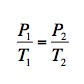The Blog

# Deflated Footballs: Mother Nature Didn't Do It

Unless you are Rip van Winkle, you are well aware that the footballs in the game in New England were underinflated. There is talk that the weather is to blame. If you run the numbers, here's what the equation looks like.
This post was published on the now-closed HuffPost Contributor platform. Contributors control their own work and posted freely to our site. If you need to flag this entry as abusive, send us an email.

Unless you are Rip van Winkle, you are well aware that the footballs in the game in New England were underinflated. There is talk that the weather is to blame. If you run the numbers, the equation looks like this:To do the calculation, let's assume that the pressure before the game is 13 psi (P), that the temperature of the locker room is 70 degrees Fahrenheit (T), and that the game was played on an unseasonably warm January day at 49 degrees Fahrenheit (T). Then, the trick is to solve for the pressure of the ball when on the field (P).

So does this drop in temperature account for the missing two pounds of pressure?

After some mathematical wrangling, the answer is no. This temperature difference between the locker room and the temperature of the field does not account for the change. You get a value of 12.5 psi, which is within the limits for the football pressure of 12.5 and 13.5 psi as set by NFL Rule 2 Section 1. (Note about the math: You can't just plug in these numbers directly, they need to be converted to Newton/meter and Kelvin and then converted back to units we use.)

Here is a simple video that does the math.

And, if we did these mathematical gymnastics again and this time used the lower limit of 12.5 psi as the starting point, then the ball pressure this time will be 12 psi.

The bottom line is that you cannot blame Mother Nature for the missing pounds of pressure, not fully.

As with science, there are of course other factors involved and more exacting ways to do this calculation. But, whether you run these numbers on back of an envelope or at a computer at NASA, the bottom line is the same:

Mother Nature is off the hook!